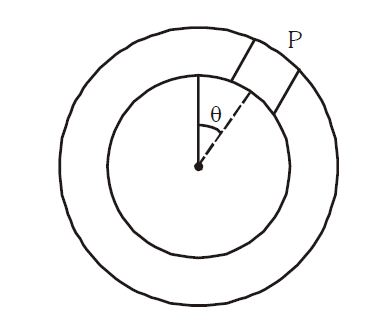# Rolling without slipping in a ring!A small block of mass $m$ is rigidly attached at $P$ to a ring of mass $3m$ and radius $r$. The system is released from rest at $\theta = 90^\circ$ and rolls without sliding.

If the angular acceleration of hoop just after release is $\large \frac{g}{nr}$, find the value of $n$.

×